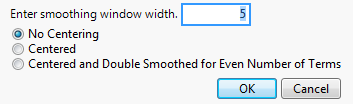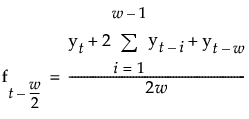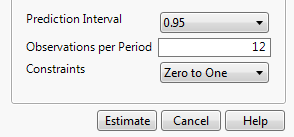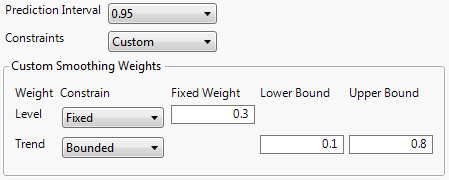Publication date: 06/16/2020

## Smoothing Model Specification Windows

### Simple Smoothing Average Specification Window

The Simple Smoothing Average Specification window appears when you select Simple Moving Average as the smoothing model. Let w be the smoothing window width in a simple moving average (SMA) model. Let ft=(yt+yt-1+yt-2+...yt-(w-2)+yt-(w-1))/w be the average of w consecutive observations for some time point t.

Figure 17.9 Simple Smoothing Average Specification WindowEnter smoothing window width

The smoothing window width, w, that defines the number of consecutive points to average. The larger the window width, the more the series is smoothed.

No Centering

The smoothing window is constructed from the points leading up to and including the time point, t, the point at which the series is being estimated. In other words, ft is the plotted value for time t.

Centered

The smoothing window is centered around the time point at which the series is being estimated.

For odd w, ft is the plotted value for time t-(w-1)/2.

For even w, ft is the plotted value for time t-(w-1)/2. When saved to a data table, ft is at t-(w-2)/2.

Centered and Double Smoothed for Even Number of Terms

For even w, the smoothing window cannot be centered around the time point at which the series is being estimated. This option creates two smoothing windows that are almost centered, and averages them together. The smoothing estimates are calculated as follows:### Smoothing Model Windows

The Smoothing Model specification windows appear when you select one of the smoothing model options other than Simple Moving Average. The title of the window and the available options depend on the smoothing model option that you select.

Figure 17.10 Smoothing Model Specification WindowPrediction Interval

Enables you to set the prediction level for the forecast prediction intervals.

Observations per Period

(Available only for seasonal smoothing models.) Enables you to set the number of observations per period in a seasonal smoothing model.

Constraints

Enables you to specify what type of constraint you want to enforce on the smoothing weights during the fit. The constraint options are as follows:

Zero To One

Constrains the values of the smoothing weights to the range zero to one.

Unconstrained

Allows the parameters to range freely.

Stable Invertible

Constrains the parameters such that the equivalent ARIMA model is stable and invertible.

Custom

Expands the dialog to enable you to set constraints on individual smoothing weights. Each smoothing weight can be Bounded, Fixed, or Unconstrained as determined by the setting of the popup menu next to the weight’s name. When entering values for fixed or bounded weights, the values can be positive or negative real numbers.

Figure 17.11 Custom Smoothing WeightsThe example shown in Figure 17.11 has the Level weight (α) fixed at a value of 0.3 and the Trend weight (γ) bounded by 0.1 and 0.8. In this case, the value of the Trend weight is allowed to move within the range 0.1 to 0.8 while the Level weight is held constant at 0.3. Note that you can specify all the smoothing weights in advance by using these custom constraints. In that case, none of the weights would be estimated from the data although forecasts and residuals would still be computed.